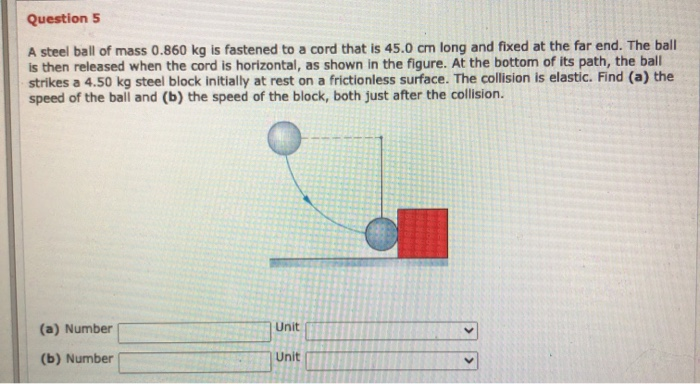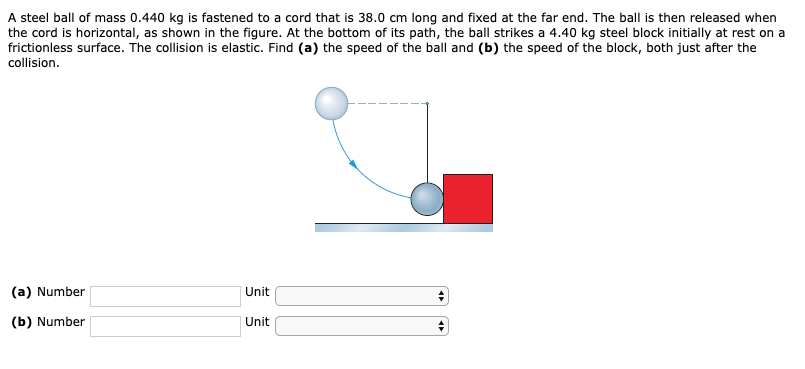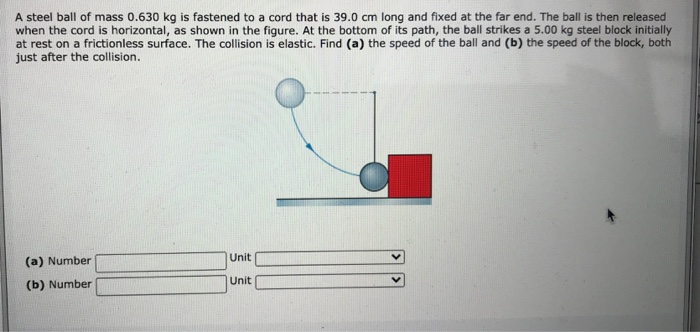# Question 5 A steel ball of mass 0.860 kg is fastened to a cord that is...Question 5 A steel ball of mass 0.860 kg is fastened to a cord that is 45.0 cm long and fixed at the far end. The ball is then released when the cord is horizontal, as shown in the figure. At the bottom of its path, the ball strikes a 4.50 kg steel block initially at rest on a frictionless surface. The collision is elastic. Find (a) the speed of the ball and (b) the speed of the block, both just after the collision. (a) Number Unit (b) Number Unit

Let the ball strike the block with a speed u1​. Since the initial speed (speed before collision) of the block =u2​=0 for the perfectly elastic collision, the speed of the block is given as
v2​=(2*m1*u1)/(m1​+m2)
where u1​ can be found by conserving energy of the ball between the position A and B before collision of m1​
⇒1/2*m1u1^2−mgl=0
u1=√(2.10*0.45)=3m/s
From this V2=(2*0.86*3)/(0.86+4.5)=0.963m/s. Is the speed of block after collision

From conservation of momentum

m1u1+m2u2=m1v1+m2v2

0.86*3=(0.86*V1)+(4.5*0.963)

V1=-2.038m/s

##### Add Answer of: Question 5 A steel ball of mass 0.860 kg is fastened to a cord that is...
Similar Homework Help Questions
• ### A steel ball of mass 0.440 kg is fastened to a cord that is 38.0 cm...A steel ball of mass 0.440 kg is fastened to a cord that is 38.0 cm long and fixed at the far end. The ball is then released when the cord is horizontal, as shown in the figure. At the bottom of its path, the ball strikes a 4.40 kg steel block initially at rest on a frictionless surface. The collision is elastic. Find (a) the speed of the ball and (b) the speed of the block, both just after...

• ### A steel ball of mass 0.630 kg is fastened to a cord that is 39.0 cm...A steel ball of mass 0.630 kg is fastened to a cord that is 39.0 cm long and fixed at the far end. The ball is then released when the cord is horizontal, as shown in the figure. At the bottom of its path, the ball strikes a 5.00 kg steel block initially at rest on a frictionless surface. The collision is elastic. Find (a) the speed of the ball and (b) the speed of the block, both just after...

• ### A steel ball of mass 0.230 kg is fastened to a cord that is 74.0 cm...A steel ball of mass 0.230 kg is fastened to a cord that is 74.0 cm long and fixed at the far end. The ball is then released when the cord is horizontal, as shown in the figure. At the bottom of its path, the ball strikes a 2.00 kg steel block initially at rest on a frictionless surface. The collision is elastic. Find (a) the speed of the ball and (b) the speed of the block, both just after...

• ### A steel ball of mass 0.600 kg is fastened to a cord that is 60.0 cm...

A steel ball of mass 0.600 kg is fastened to a cord that is 60.0 cm long and fixed at the far end. The ball is then released when the cord is horizontal. At the bottom of its path, the ball strikes a 3.00 kg steel block initially at rest on a frictionless surface. The collision is elastic. (a) Find the speed of the ball just after collision. ( in m/s) (b) Find the speed of the block just after...

• ### A steel ball of mass 0.470 kg is fastened to a cord that is 92.0 cm long and fixed at the far end. The ball is then rele...

A steel ball of mass 0.470 kg is fastened to a cord that is 92.0 cm long and fixed at the far end. The ball is then released when the cord is horizontal, as shown in the figure. At the bottom of its path, the ball strikes a 2.90 kg steel block initially at rest on a frictionless surface. The collision is elastic. Find (a) the speed of the ball and (b) the speed of the block, both just after...

• ### a steel ball

A steel ball of mass 0.880 kg is fastened to a cord that is 59.0 cm long and fixed at the far end. The ball is then released when the cord is horizontal, as shownin Fig. 9-65. At the bottom of its path, the ball strikes a 2.40 kg steel block initially at rest on a frictionless surface. The collision is elastic. Find (a) thespeed of the ball and (b) the speed of the block, both just after the collision.

• ### ball on string

A steel ball of mass 0.600 kg is fastened to a cord that is 80.0 cm long and fixed at the far end. The ball is then released when the cord is horizontal. At the bottomof its path, the ball strikes a 2.00 kg steel block initially at rest on a frictionless surface. The collision is elastic.a) Find the speed of the ball just after collision.(b) Find the speed of the block just after collision.

• ### Center of Mass and Linear Momentum..A steel ball of mass 0.380 kg is fastened to a cord that is42.0 cm long and fixed at the far end. The ball is then releasedwhen the cord is horizontal (Fig. 9-68).At the bottom of its path,the ball strikes a 4.50 kg steel block initially at rest on africtionless surface. The collision is elastic. Find(a) thespeed of the ball and (b)the speed of the block, both just after the collision.

• ### ball on a string

A steel ball of mass 0.600 kg is fastened to a cord that is 80.0 cm long and fixed at the far end. The ball is then released when the cord is horizontal. At the bottomof its path, the ball strikes a 2.00 kg steel block initially at rest on a frictionless surface. The collision is elastic.(a) Find the speed of the ball just after collision.m/s(b) Find the speed of the block just after collision.m/s

• ### finding speed of ball and block in an elastic collision

A steel ball of mass 0.400 kg is fastened to a cord that is 60.0 cm long and fixed at the far end. The ball is then released when the cord is horizontal. At the bottomof its path, the ball strikes a 3.00 kg steel block initially at rest on a frictionless surface. The collision is elastic.(a) Find the speed of the ball just after collision.m/s(b) Find the speed of the block just after collision.m/s

Free Homework App